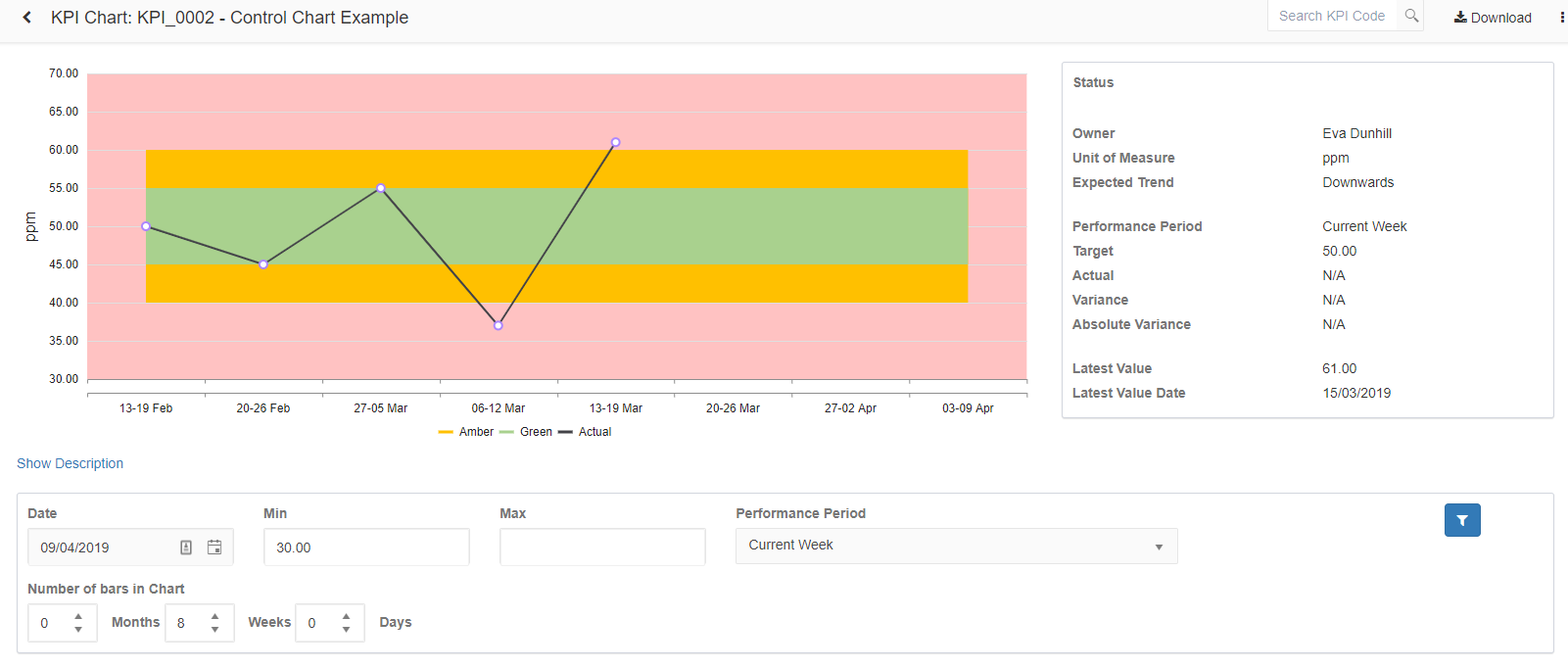To display a controlled KPI chart similar to the one displayed below. Note that the Regions are determined based on the specified bound values with the captured targets.The KPI above has been configured as follows on the Edit screen.The Target value used for this example is 50, as a result, the Green and Amber regions will be determined as follows:

Green Region:

Lower Green bound will be  = Target value - Lower bound Value = 50 - 5 = 45 as a Lower green bound.

Upper Green bound will be  = Target value + Upper bound Value = 50 + 5 = 55 as a Upper green bound.

Amber Region (This values should not be less than that of the Green bound region):

Lower Amber bound will be  = Target value - Lower bound Value = 50 - 10 = 40 as a Lower Amber bound.

Upper Amber bound will be  = Target value + Upper bound Value = 50 + 10 = 60 as a Upper Amber bound.

Further note, the displayed region changes will the captured Target Value, a specified Actual will then be plotted under the relevant region green or amber otherwise it will be displayed outside the defined region.